CS 007 -- Modular Arithmetic, Handout 2

copyright Philip Klein, 1996

Let's define the modulus to be 7; by that I mean we are going to do arithmetic ``modulo 7''.

This defines funny glasses through which certain differences between numbers are blurred. For example, the numbersall appear to be the same number. Similarly,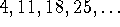are all the same. When the modulus is 7, in fact, there appear to be only seven numbers in the whole world. For each of these numbers, there are several names; for example, 1,8,15, and 22 are all names of the same number. However, for convenience we typically distinguish one standard name for each number, the smallest nonnegative name for that number. That is, the standard names modulo 7 are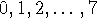.

We say two numbers are congruent (modulo 7) if they look the same to someone wearing modulo-7 glasses. For example, 1 and 8 are congruent (modulo 7), and 3 is congruent (modulo 7) to 10 and to 17. If we want to be real math-y, we can use mathematical notation for ``congruent (modulo 7)''. One way to write that 1 and 8 are congruent (modulo 7) isFinally, we sometimes get tired of writing ``modulo 7'' over and over. If (as often occurs) we are doing lots of arithmetic with the same modulus, we can just say up front that the modulus is, for example, 7, and then not mention it again! Thus it is okay to say ``1 is congruent to 8'' if everybody already knows the modulus is 7.

How can you determine for a big number like 436 what the standard name is? You compute the remainder when 436 is divided by 7. For example, in this case 436 is 7 times 62 plus 2, so the remainder is 2. Thus the standard name of 436 is 2. Try another: since 65 is 7 times 9 plus 2, the standard name of 65 is also 2. We can therefore assert that 436 and 65 are congruent (modulo 7).

Computing the remainder is an arithmetic operation like + and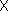, so we should have some notation for writing the operation. Just as we write 2+3 for the sum of 2 and 3, we write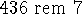for the remainder when 436 is divided by 7. That is,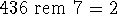.

More examples: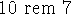is 3, and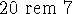is 6. What is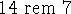? Zero! What is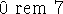? Zero again! (What about negatives? What is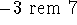? Uh, well, that's 4. The remainder is by definition always greater than or equal to zero.)

How can you tell if two numbers are congruent (modulo 7)? For example, are 123 and 802 congruent? One good way to check this is to compute the remainders: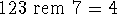and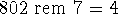(unless I've made a mistake), so 123 and 802 are indeed congruent (modulo 7).

Note that if you start with any number (say 633) and add a multiple of 7 to it (say 3 times 7, which is 21) you get a number (654 in this case) that is guaranteed to be congruent to the number you started with. Thus adding a multiple of the modulus doesn't change the number. It's as if you added zero. Well, actually you did: note that a multiple of 7 is congruent to zero: 0,7,14,21, ...are all different names for the same number. Since that number's standard name is zero, it's not surprising that it acts like zero in that adding it to something else doesn't really change the something else.

One more thing about modular addition. Because we interpret different numbers as if they were different names for the same number, you can sometimes simplify your work by replacing a number with its standard name. Suppose we want to add up the following numbers (modulo 7):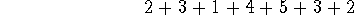Well, 2+3 is of course 5, and adding 1 gives 6. Then we add 4, which gives 10. But 10 is the same as 3, so let's call it 3. Now we add 5 to 3, yielding 8, which is the same as 1. Now we add 3 to 1, getting 4. Finally, we add 2 to 4, getting 6.

Summary:

• ``rem'' means ``remainder when divided by'' (except for negative numbers)
``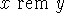'' means ``remainder when x is divided by y''
• To determine whether two numbers are congruent (modulo 7), find their remainders when divided by 7.
``x is congruent (modulo 7) to y'' is true if ``x rem 7 = y rem 7''
• Modulo 7, the standard name of a number x is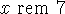.
• When doing addition (modulo 7), you can at any time replace a number by another of its names, e.g. its standard name.
• When we ask you to give a result of modular arithmetic in standard form, we mean give the standard name of the answer.

Lisa Eckstein
Tue Sep 17 04:35:49 EDT 1996